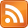# kaashif's blog

Programming, software freedom and Unix### 2014-02-05

Whenever you hear something about Haskell, chances are it sounds arcane and involves lots of complicated and intimidating mathematical language. Well, the truth is that all this talk of 'endofunctors' and 'monoids' is really unnecessary, if the concept of functors is explained using a simple analogy.

If you have a situation where a type contains other types, you are dealing with a functor. For example, when you have a list of integers, the list is the functor. If you have a herd of sheep, the herd is the functor. At its core, this is what a functor is - something which contains types and can be mapped over. That last part is crucial and is central to how functors are defined in Haskell, but are not central to how one should think about functors.

## In practice

At a GHCi prompt, you can import a few modules here and there with the word 'functor' in them, but at this stage, you do not require any of the more complex functions, you only need one - fmap. The functor we'll be using as an example is the 'Maybe' functor, which contains either a single value ('Just' the value) or Nothing. If this is confusing to you, just think of the 'Just' constructor as representing a box you put types into, and the 'Nothing' constructor as representing an empty box. So 'Just 1' can be represented as 'put the value 1 into a box'. This isn't entirely contrived, the 'Maybe' functor does have a use in functions that can fail, so can return an empty box or a box with a result in them.

Let's examine the type of 'Just' using ':t'.

Prelude> :t Just
Just :: a -> Maybe a


If you are familiar with Haskell syntax, you should know that this means that 'Just' takes any type and turns it into a 'Maybe' functor containing that type. No surprises here, that is the definition of a functor.

Let's say we have a few functions that return numbers contained in a Maybe functor, and we want to combine them in some way. When mapping over a single functor, the function you use is fmap, like so:

Prelude> fmap (+2) (Just 3)
Just 5


This is fine for function that only take 1 argument, but remember, we want to apply this to multiple arguments. If we define a function like so, which takes three (non-Maybe) numbers and adds them together, it becomes obvious that using fmap on its own won't work:

Prelude> let f x y z = x*y*z


If we just try to fmap our three-operand function over a Maybe functor, and examine its type, we see the following:

Prelude> :t (fmap (f) (Just 3))
(fmap (f) (Just 3)) :: Num a => Maybe (a -> a -> a)


This means that fmap has transformed our function which took things of typeclass Num into a partially applied function, which still takes things of typeclass Num, but the function itself is within the box of the Maybe functor. This means that we want to map a Maybe function over a Maybe Num. If the function takes yet another argument, doing this will return yet another function. If not, it'll give us the answer, wrapped in a functor. If we want to apply a function with multiple arguments to functors, we need to use Applicative functors.

Prelude> import Control.Applicative


And then, we can use the <*> operator, which takes a function in a functor and applies it to a value in a functor. We can use it in more trivial cases like so:

Prelude> Just (*4) <*> Just 3
Just 12


We can see that (*4) takes one value, so we are given the answer we want. Applying this to our situation gives us this:

Prelude> (fmap (f) (Just 3)) <*> Just 4 <*> Just 5
Just 60


We can improve the look of this ugly statement by using fmap as an infix function.

Prelude> f fmap Just 3 <*> Just 4 <*> Just 5


Because this is such a common use case, there is some syntactic sugar provided - fmap can be replaced with <$>. Prelude> f <$> Just 3 <*> Just 4 <*> Just 5


At this point, we just have the function and its arguments as we usually would, just with some extra operators thrown in. The most important thing you should take away from this is that using Applicative is far better than using liftM and its numbered equivalents, as Applicative can be applied to an arbitrary number of arguments. Monads are useful in other cases, and Maybe is actually a monad, but thinking about it like a monad rarely helps.